Скачать презентацию Efficient Contention Resolution Protocols for Selfish Agents Amos

• Количество слайдов: 33Efficient Contention Resolution Protocols for Selfish Agents Amos Fiat, Joint work with Yishay Mansour and Uri Nadav Tel-Aviv University, Israel Workshop on Algorithmic Game Theory, University of Warwick, UK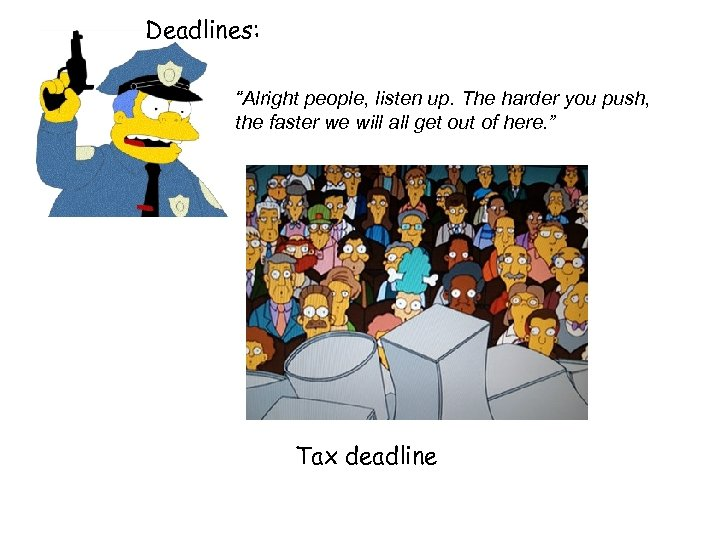Deadlines: “Alright people, listen up. The harder you push, the faster we will all get out of here. ” Tax deadline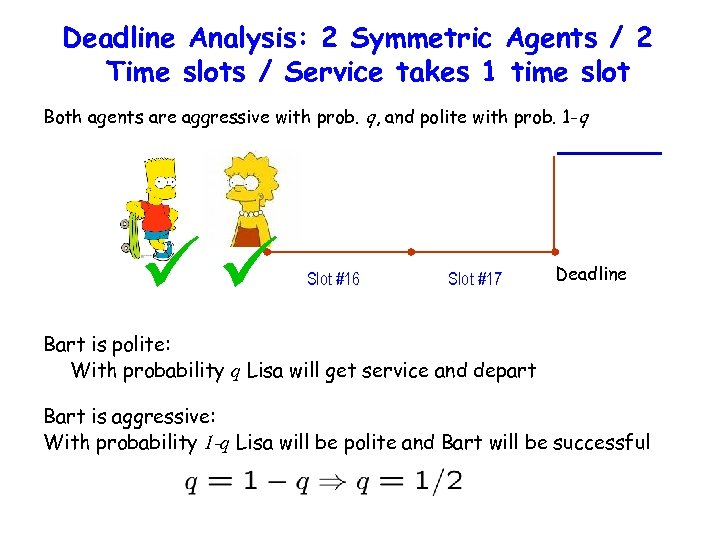Deadline Analysis: 2 Symmetric Agents / 2 Time slots / Service takes 1 time slot Both agents are aggressive with prob. q, and polite with prob. 1 -q Slot #16 Slot #17 Deadline Bart is polite: With probability q Lisa will get service and depart Bart is aggressive: With probability 1 -q Lisa will be polite and Bart will be successful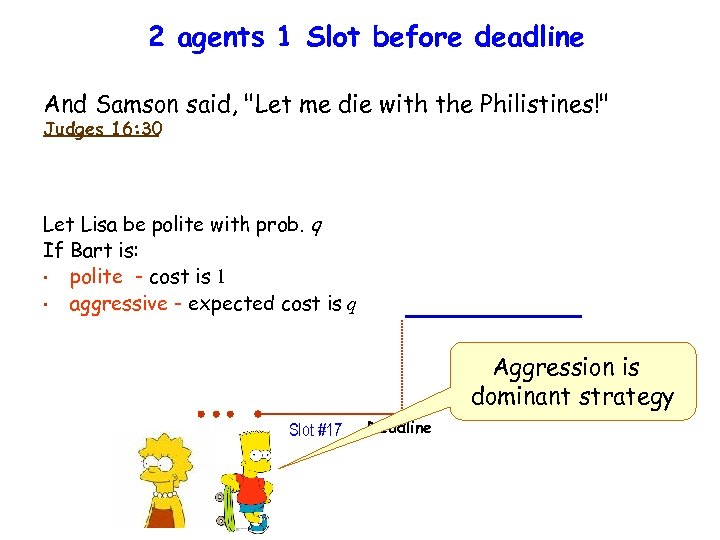2 agents 1 Slot before deadline And Samson said, "Let me die with the Philistines!" Judges 16: 30 Let Lisa be polite with prob. q If Bart is: • polite - cost is 1 • aggressive - expected cost is q Aggression is dominant strategy Slot #17 Deadline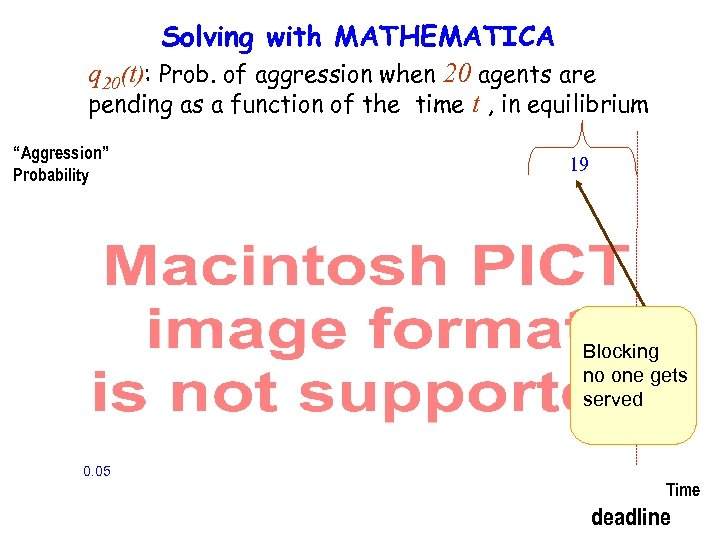Solving with MATHEMATICA q 20(t): Prob. of aggression when 20 agents are pending as a function of the time t , in equilibrium “Aggression” Probability 19 Blocking no one gets served 0. 05 Time deadline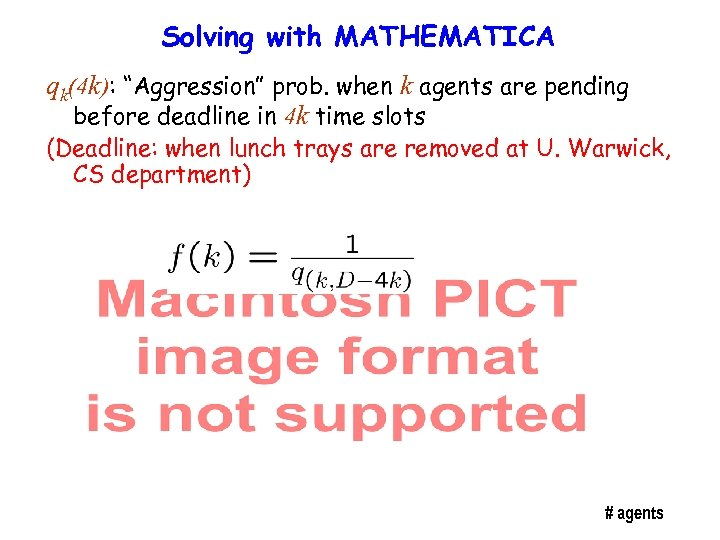Solving with MATHEMATICA qk(4 k): “Aggression” prob. when k agents are pending before deadline in 4 k time slots (Deadline: when lunch trays are removed at U. Warwick, CS department) # agents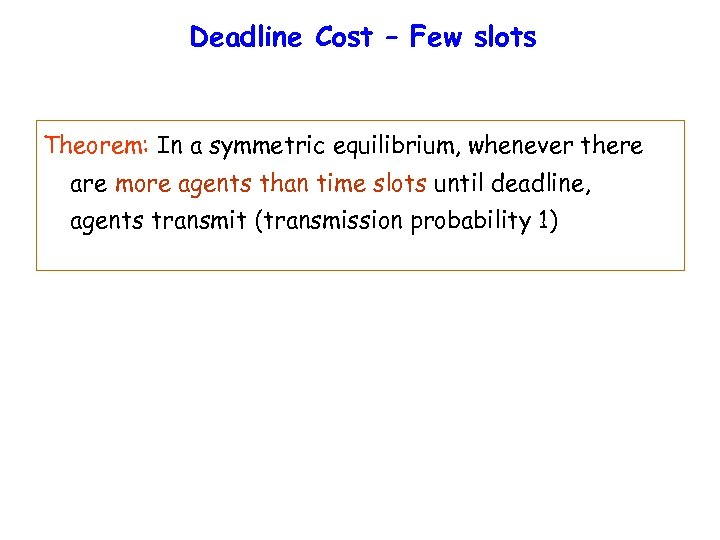Deadline Cost – Few slots Theorem: In a symmetric equilibrium, whenever there are more agents than time slots until deadline, agents transmit (transmission probability 1)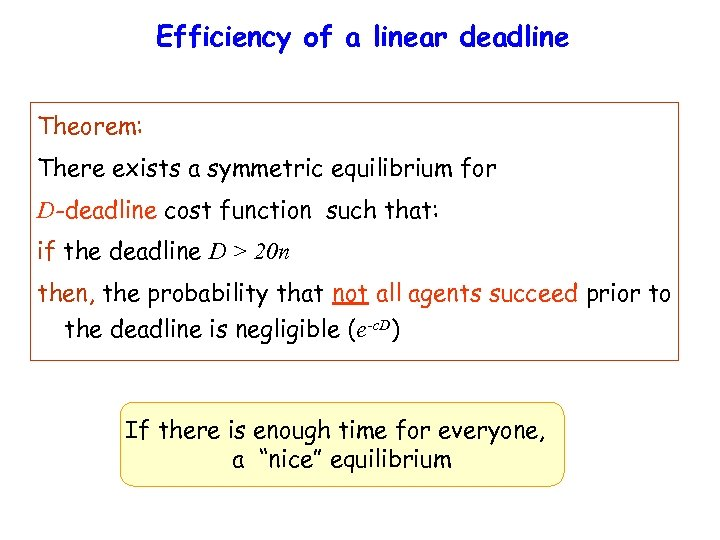Efficiency of a linear deadline Theorem: There exists a symmetric equilibrium for D-deadline cost function such that: if the deadline D > 20 n then, the probability that not all agents succeed prior to the deadline is negligible (e-c. D) If there is enough time for everyone, a “nice” equilibrium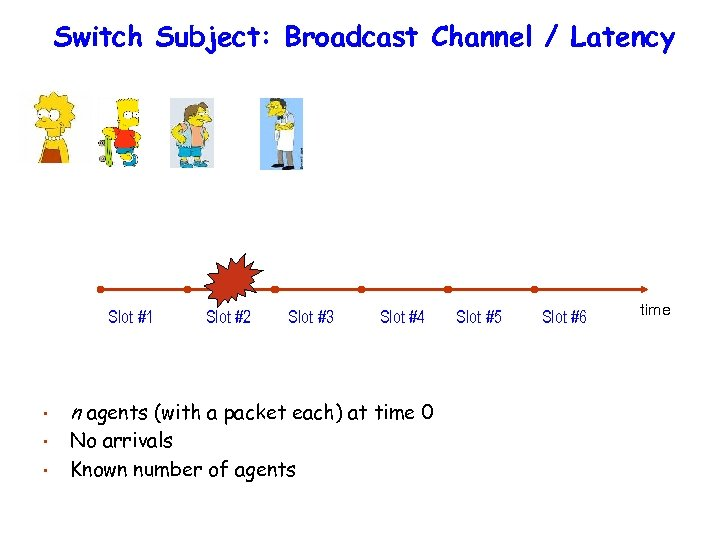Switch Subject: Broadcast Channel / Latency Slot #1 Slot #2 Slot #3 Slot #4 • n agents (with a packet each) at time 0 • No arrivals Known number of agents • Slot #5 Slot #6 time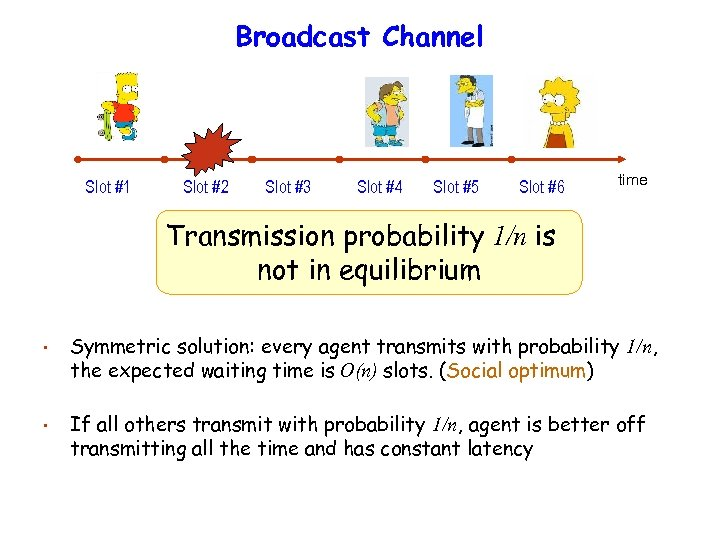Broadcast Channel Slot #1 Slot #2 Slot #3 Slot #4 Slot #5 Slot #6 time Transmission probability 1/n is not in equilibrium • Symmetric solution: every agent transmits with probability 1/n, the expected waiting time is O(n) slots. (Social optimum) • If all others transmit with probability 1/n, agent is better off transmitting all the time and has constant latency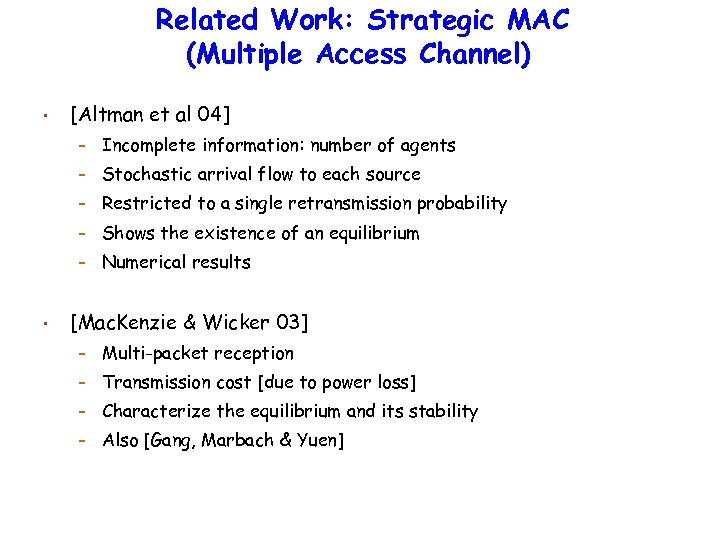Related Work: Strategic MAC (Multiple Access Channel) • [Altman et al 04] – – Stochastic arrival flow to each source – Restricted to a single retransmission probability – Shows the existence of an equilibrium – • Incomplete information: number of agents Numerical results [Mac. Kenzie & Wicker 03] – Multi-packet reception – Transmission cost [due to power loss] – Characterize the equilibrium and its stability – Also [Gang, Marbach & Yuen]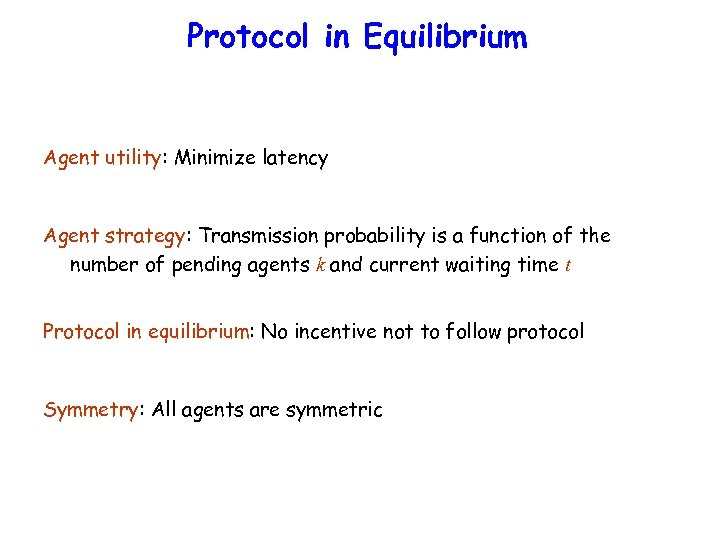Protocol in Equilibrium Agent utility: Minimize latency Agent strategy: Transmission probability is a function of the number of pending agents k and current waiting time t Protocol in equilibrium: No incentive not to follow protocol Symmetry: All agents are symmetric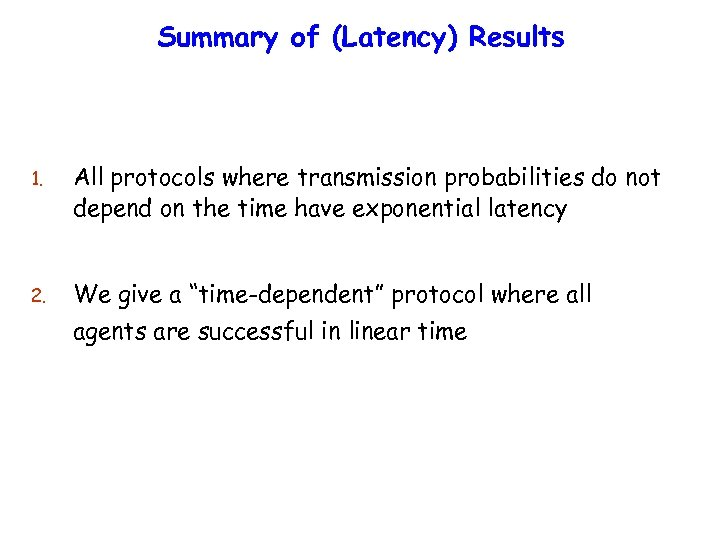Summary of (Latency) Results 1. All protocols where transmission probabilities do not depend on the time have exponential latency 2. We give a “time-dependent” protocol where all agents are successful in linear time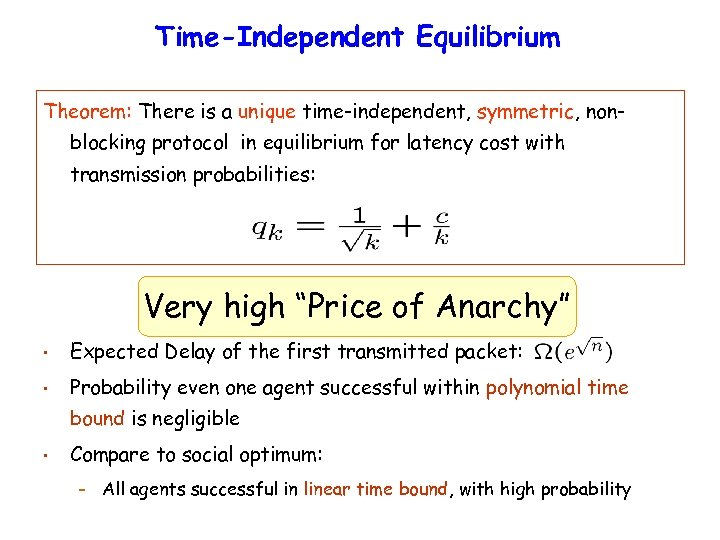Time-Independent Equilibrium Theorem: There is a unique time-independent, symmetric, nonblocking protocol in equilibrium for latency cost with transmission probabilities: Very high “Price of Anarchy” • Expected Delay of the first transmitted packet: • Probability even one agent successful within polynomial time bound is negligible • Compare to social optimum: – All agents successful in linear time bound, with high probability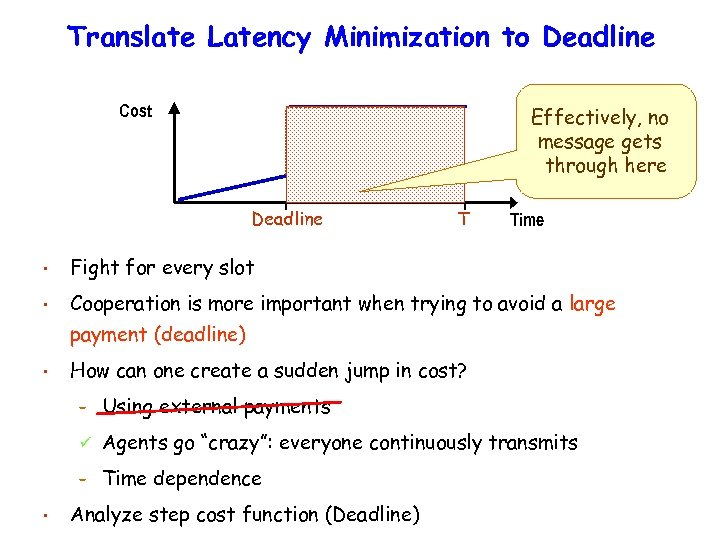Translate Latency Minimization to Deadline Cost Effectively, no message gets through here Deadline T Time • Fight for every slot • Cooperation is more important when trying to avoid a large payment (deadline) • How can one create a sudden jump in cost? – Agents go “crazy”: everyone continuously transmits – • Using external payments Time dependence Analyze step cost function (Deadline)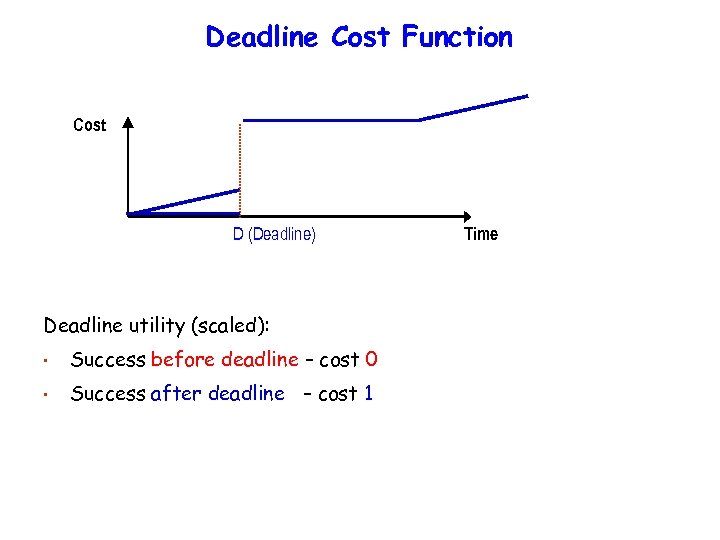Deadline Cost Function Cost D (Deadline) Deadline utility (scaled): • Success before deadline – cost 0 • Success after deadline – cost 1 Time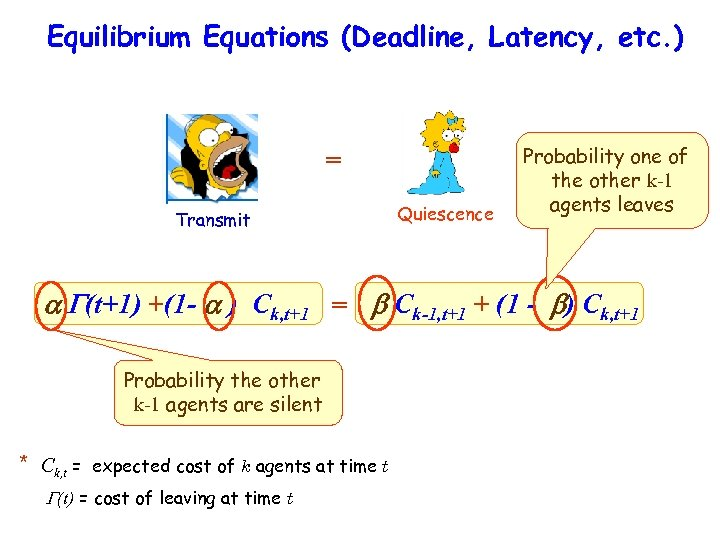Equilibrium Equations (Deadline, Latency, etc. ) = Transmit Quiescence Probability one of the other k-1 agents leaves (t+1) +(1 - ) Ck, t+1 = Ck-1, t+1 + (1 - ) Ck, t+1 Probability the other k-1 agents are silent * Ck, t = expected cost of k agents at time t (t) = cost of leaving at time t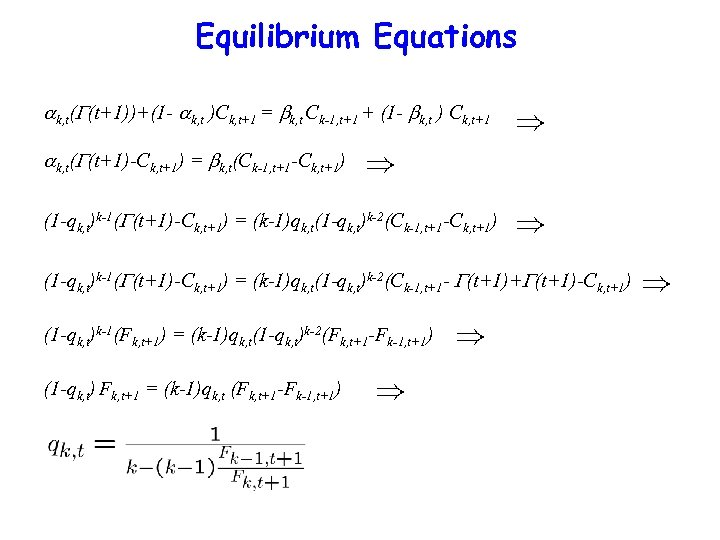Equilibrium Equations k, t( (t+1))+(1 - k, t )Ck, t+1 = k, t Ck-1, t+1 + (1 - k, t ) Ck, t+1 k, t( (t+1)-Ck, t+1) = k, t(Ck-1, t+1 -Ck, t+1) (1 -qk, t)k-1( (t+1)-Ck, t+1) = (k-1)qk, t(1 -qk, t)k-2(Ck-1, t+1 - (t+1)+ (t+1)-Ck, t+1) (1 -qk, t)k-1(Fk, t+1) = (k-1)qk, t(1 -qk, t)k-2(Fk, t+1 -Fk-1, t+1) (1 -qk, t) Fk, t+1 = (k-1)qk, t (Fk, t+1 -Fk-1, t+1)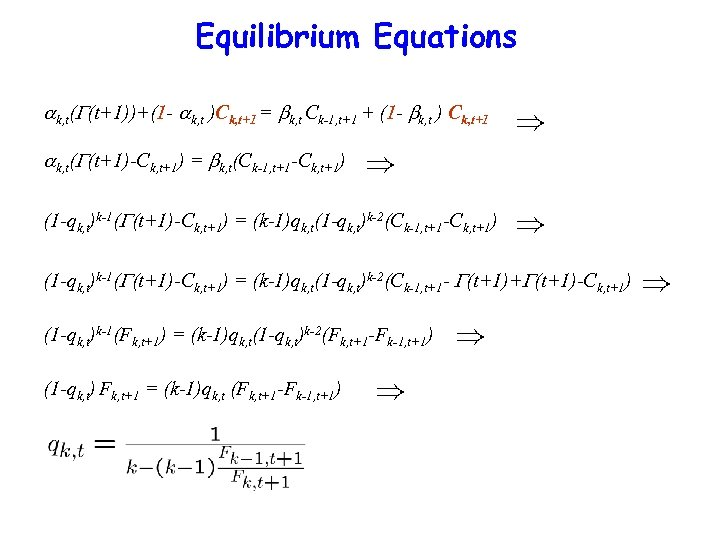Equilibrium Equations k, t( (t+1))+(1 - k, t )Ck, t+1 = k, t Ck-1, t+1 + (1 - k, t ) Ck, t+1 k, t( (t+1)-Ck, t+1) = k, t(Ck-1, t+1 -Ck, t+1) (1 -qk, t)k-1( (t+1)-Ck, t+1) = (k-1)qk, t(1 -qk, t)k-2(Ck-1, t+1 - (t+1)+ (t+1)-Ck, t+1) (1 -qk, t)k-1(Fk, t+1) = (k-1)qk, t(1 -qk, t)k-2(Fk, t+1 -Fk-1, t+1) (1 -qk, t) Fk, t+1 = (k-1)qk, t (Fk, t+1 -Fk-1, t+1)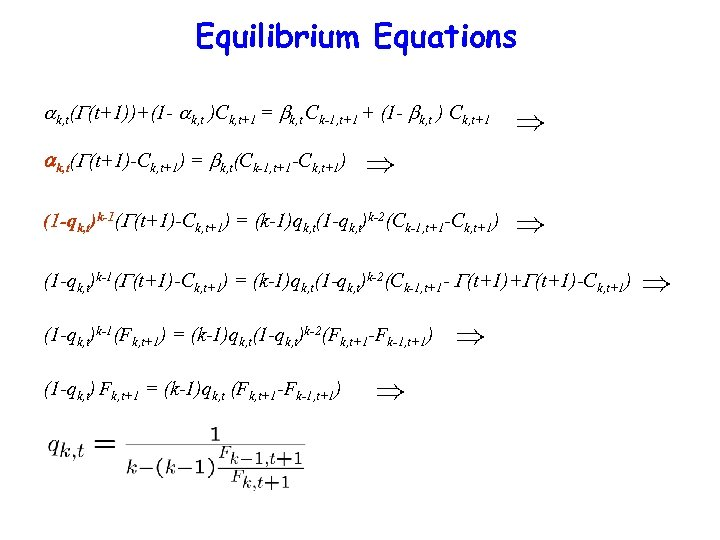Equilibrium Equations k, t( (t+1))+(1 - k, t )Ck, t+1 = k, t Ck-1, t+1 + (1 - k, t ) Ck, t+1 k, t( (t+1)-Ck, t+1) = k, t(Ck-1, t+1 -Ck, t+1) (1 -qk, t)k-1( (t+1)-Ck, t+1) = (k-1)qk, t(1 -qk, t)k-2(Ck-1, t+1 - (t+1)+ (t+1)-Ck, t+1) (1 -qk, t)k-1(Fk, t+1) = (k-1)qk, t(1 -qk, t)k-2(Fk, t+1 -Fk-1, t+1) (1 -qk, t) Fk, t+1 = (k-1)qk, t (Fk, t+1 -Fk-1, t+1)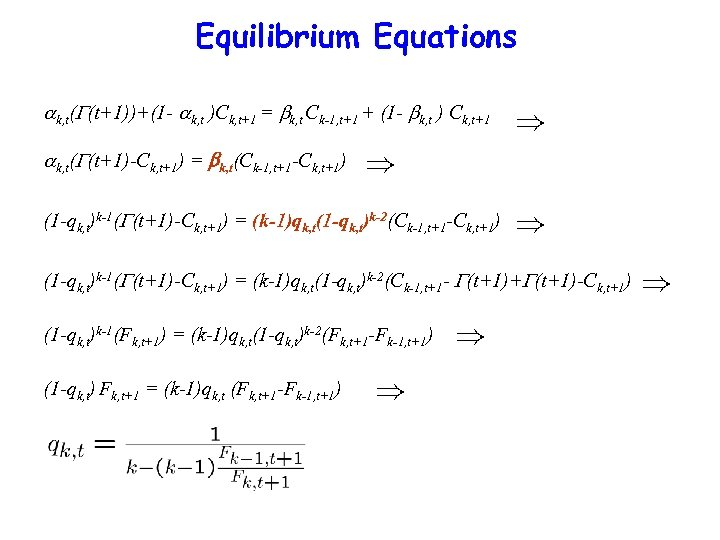Equilibrium Equations k, t( (t+1))+(1 - k, t )Ck, t+1 = k, t Ck-1, t+1 + (1 - k, t ) Ck, t+1 k, t( (t+1)-Ck, t+1) = k, t(Ck-1, t+1 -Ck, t+1) (1 -qk, t)k-1( (t+1)-Ck, t+1) = (k-1)qk, t(1 -qk, t)k-2(Ck-1, t+1 - (t+1)+ (t+1)-Ck, t+1) (1 -qk, t)k-1(Fk, t+1) = (k-1)qk, t(1 -qk, t)k-2(Fk, t+1 -Fk-1, t+1) (1 -qk, t) Fk, t+1 = (k-1)qk, t (Fk, t+1 -Fk-1, t+1)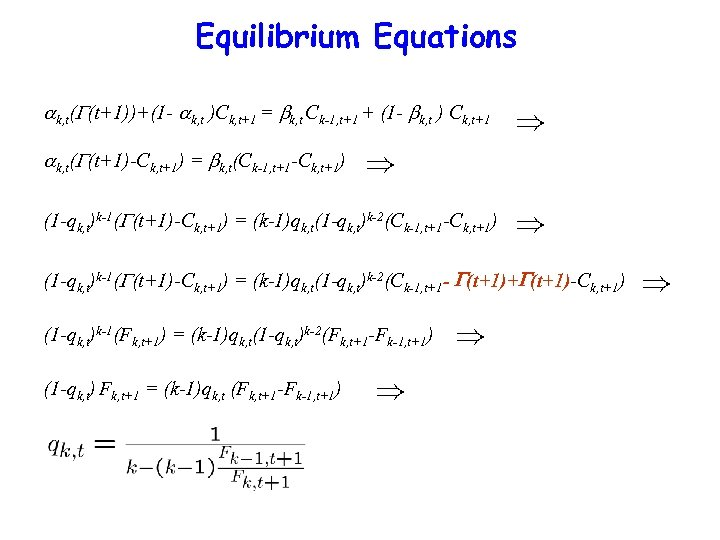Equilibrium Equations k, t( (t+1))+(1 - k, t )Ck, t+1 = k, t Ck-1, t+1 + (1 - k, t ) Ck, t+1 k, t( (t+1)-Ck, t+1) = k, t(Ck-1, t+1 -Ck, t+1) (1 -qk, t)k-1( (t+1)-Ck, t+1) = (k-1)qk, t(1 -qk, t)k-2(Ck-1, t+1 - (t+1)+ (t+1)-Ck, t+1) (1 -qk, t)k-1(Fk, t+1) = (k-1)qk, t(1 -qk, t)k-2(Fk, t+1 -Fk-1, t+1) (1 -qk, t) Fk, t+1 = (k-1)qk, t (Fk, t+1 -Fk-1, t+1)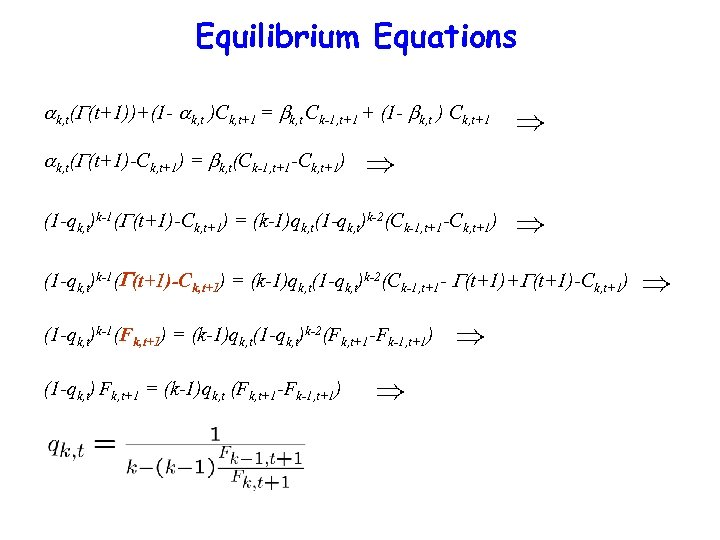Equilibrium Equations k, t( (t+1))+(1 - k, t )Ck, t+1 = k, t Ck-1, t+1 + (1 - k, t ) Ck, t+1 k, t( (t+1)-Ck, t+1) = k, t(Ck-1, t+1 -Ck, t+1) (1 -qk, t)k-1( (t+1)-Ck, t+1) = (k-1)qk, t(1 -qk, t)k-2(Ck-1, t+1 - (t+1)+ (t+1)-Ck, t+1) (1 -qk, t)k-1(Fk, t+1) = (k-1)qk, t(1 -qk, t)k-2(Fk, t+1 -Fk-1, t+1) (1 -qk, t) Fk, t+1 = (k-1)qk, t (Fk, t+1 -Fk-1, t+1)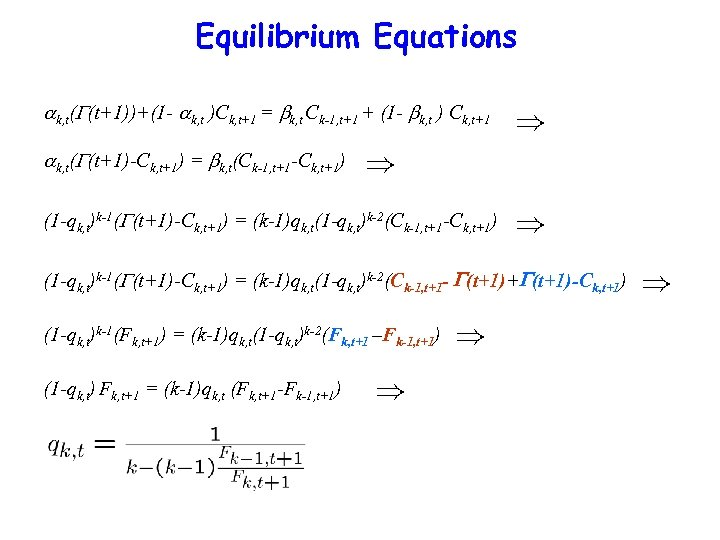Equilibrium Equations k, t( (t+1))+(1 - k, t )Ck, t+1 = k, t Ck-1, t+1 + (1 - k, t ) Ck, t+1 k, t( (t+1)-Ck, t+1) = k, t(Ck-1, t+1 -Ck, t+1) (1 -qk, t)k-1( (t+1)-Ck, t+1) = (k-1)qk, t(1 -qk, t)k-2(Ck-1, t+1 - (t+1)+ (t+1)-Ck, t+1) (1 -qk, t)k-1(Fk, t+1) = (k-1)qk, t(1 -qk, t)k-2(Fk, t+1 –Fk-1, t+1) (1 -qk, t) Fk, t+1 = (k-1)qk, t (Fk, t+1 -Fk-1, t+1)Equilibrium Equations k, t( (t+1))+(1 - k, t )Ck, t+1 = k, t Ck-1, t+1 + (1 - k, t ) Ck, t+1 k, t( (t+1)-Ck, t+1) = k, t(Ck-1, t+1 -Ck, t+1) (1 -qk, t)k-1( (t+1)-Ck, t+1) = (k-1)qk, t(1 -qk, t)k-2(Ck-1, t+1 - (t+1)+ (t+1)-Ck, t+1) (1 -qk, t)k-1(Fk, t+1) = (k-1)qk, t(1 -qk, t)k-2(Fk, t+1 -Fk-1, t+1) (1 -qk, t) Fk, t+1 = (k-1)qk, t (Fk, t+1 -Fk-1, t+1)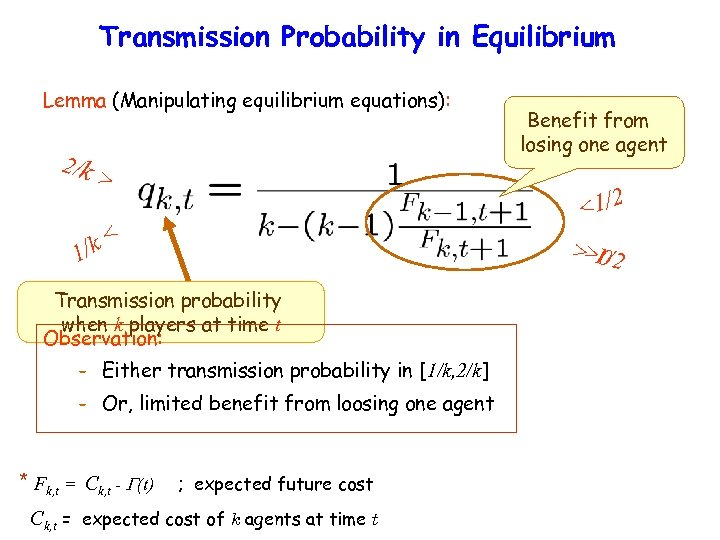Transmission Probability in Equilibrium Lemma (Manipulating equilibrium equations): 2/k > <1/2 < /k 1 > 1/2 >0 Transmission probability when k players at time t Observation: – Either transmission probability in [1/k, 2/k] – Or, limited benefit from loosing one agent * Fk, t = Ck, t - (t) Benefit from losing one agent ; expected future cost Ck, t = expected cost of k agents at time t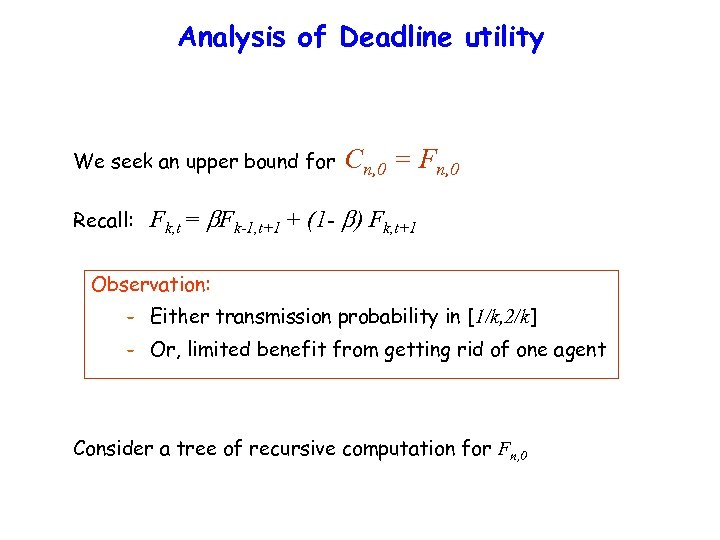Analysis of Deadline utility We seek an upper bound for Recall: Cn, 0 = Fn, 0 Fk, t = Fk-1, t+1 + (1 - ) Fk, t+1 Observation: – Either transmission probability in [1/k, 2/k] – Or, limited benefit from getting rid of one agent Consider a tree of recursive computation for Fn, 0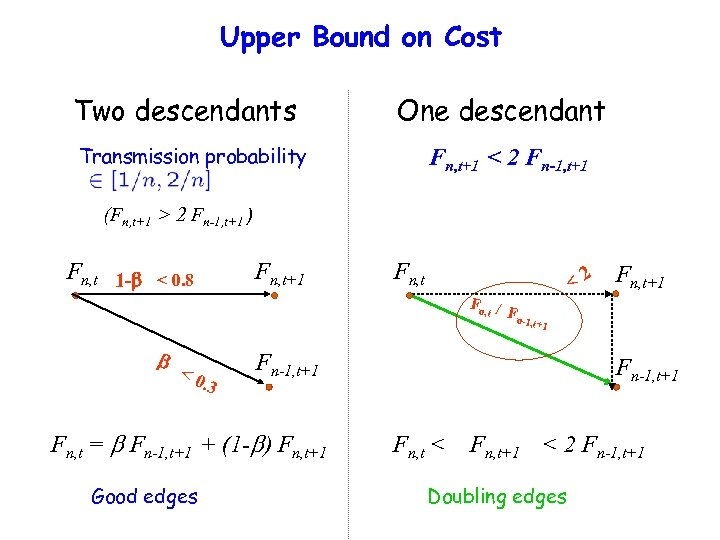Upper Bound on Cost Two descendants One descendant Transmission probability Fn, t+1 < 2 Fn-1, t+1 (Fn, t+1 > 2 Fn-1, t+1 ) Fn, t 1 - Fn, t+1 < 0. 8 Fn, t <2 Fn, / t Fn- Fn, t+1 1, t+1 <0 . 3 Fn-1, t+1 Fn, t = Fn-1, t+1 + (1 - ) Fn, t+1 Good edges Fn-1, t+1 Fn, t < Fn, t+1 < 2 Fn-1, t+1 Doubling edges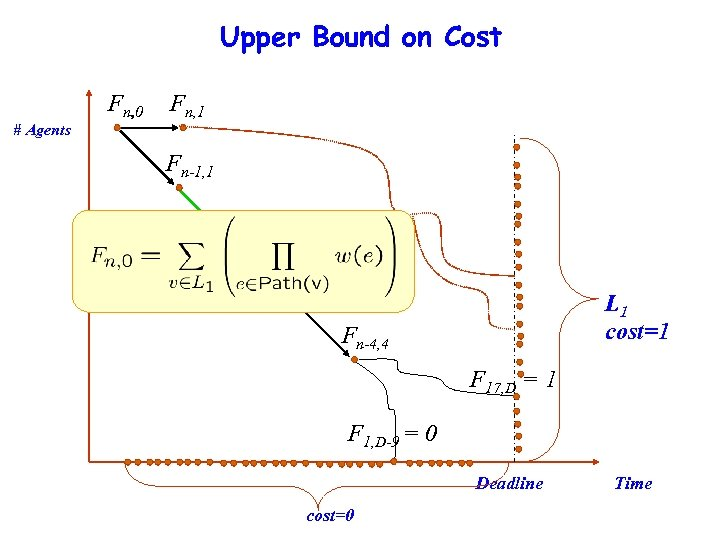Upper Bound on Cost Fn, 0 Fn, 1 # Agents Fn-1, 1 Fn-2, 2 Fn-3, 3 F n-3, 4 L 1 cost=1 Fn-4, 4 F 17, D = 1 F 1, D-9 = 0 Deadline cost=0 Time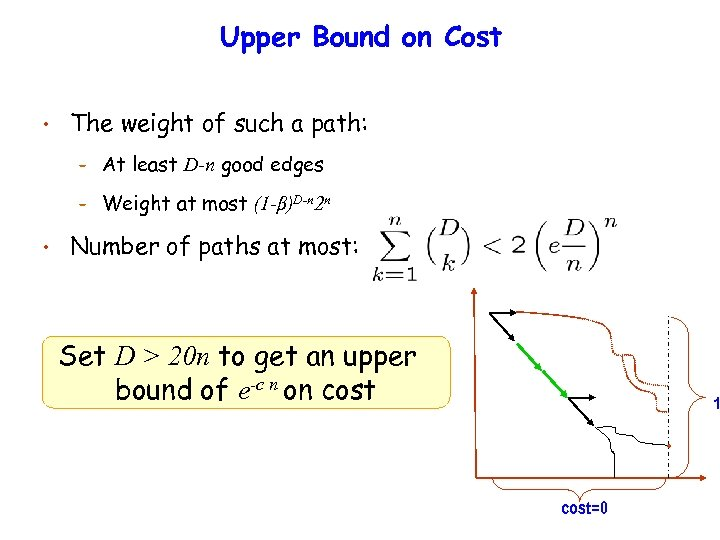Upper Bound on Cost • The weight of such a path: – – • At least D-n good edges Weight at most (1 -β)D-n 2 n Number of paths at most: Set D > 20 n to get an upper bound of e-c n on cost 1 cost=0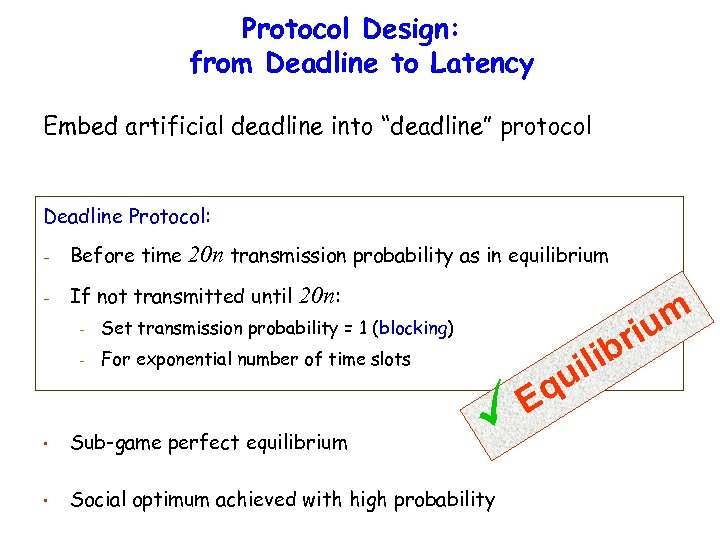Protocol Design: from Deadline to Latency Embed artificial deadline into “deadline” protocol Deadline Protocol: - Before time 20 n transmission probability as in equilibrium - If not transmitted until 20 n: - um ri Set transmission probability = 1 (blocking) For exponential number of time slots • Sub-game perfect equilibrium • Social optimum achieved with high probability Eq lib ui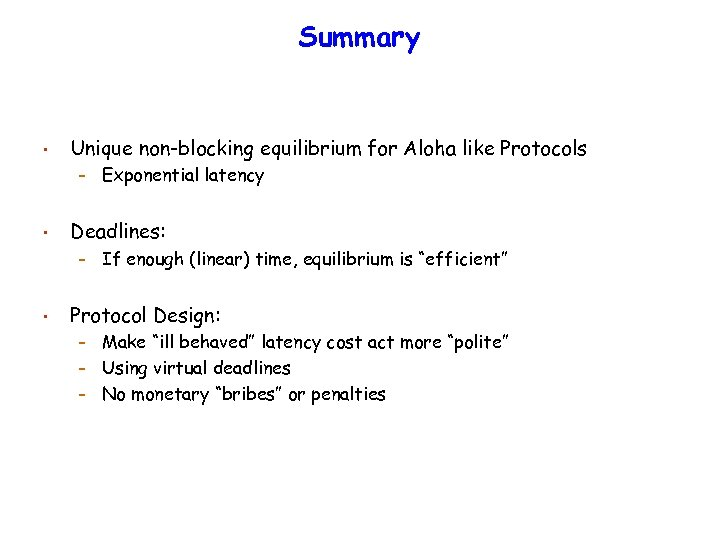Summary • Unique non-blocking equilibrium for Aloha like Protocols – • Deadlines: – • Exponential latency If enough (linear) time, equilibrium is “efficient” Protocol Design: Make “ill behaved” latency cost act more “polite” – Using virtual deadlines – No monetary “bribes” or penalties –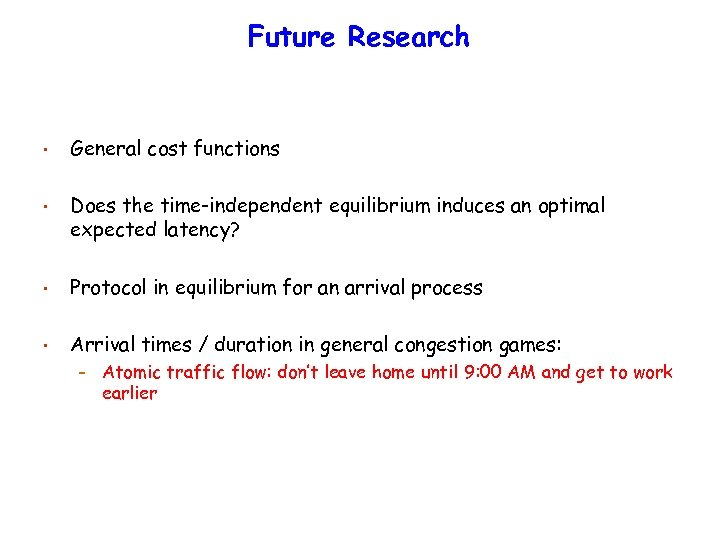Future Research • General cost functions • Does the time-independent equilibrium induces an optimal expected latency? • Protocol in equilibrium for an arrival process • Arrival times / duration in general congestion games: – Atomic traffic flow: don’t leave home until 9: 00 AM and get to work earlier